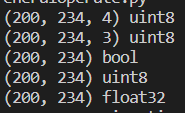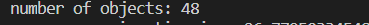0

# 《Python 计算机视觉编程》学习笔记（一）

《Python计算机视觉编程》

## 1.1 PIL： Python图像处理类库

PIL（ Python Imaging Library Python，图像处理类库）提供了通用的图像处理功能，以及大量有用的基本图像操作。

### 图像读取、显示、显示对应灰度图

from PIL import Image
from time import*defmain():# 读取一幅图像
pil_im = Image.open('empire.jpg')# 读取一幅图像，并将其转换成灰度图像，
pil_imL = Image.open('empire.jpg').convert('L')
pil_im.show()
pil_imL.show()returnif __name__ =='__main__':

timeStart = time()
main()
timeOver = time()print("program running time is :",timeOver - timeStart)### 更改图像格式（后缀）

defchangeFormat():"""
函数功能：修改图像格式
参数说明：无
函数返回：无
"""

filelist = get_imlist(r"D:\python\exercise_data\ComputerVision\ch01\figure")# 遍历整个文件夹查找出路径中符合条件的图像文件for infile in filelist:# 对原图像文件的格式进行修改
outfile = os.path.splitext(infile)+'.png'# 判断是否修改成功，成功则进行save()方法if infile !=outfile:try:
Image.open(infile).save(outfile)except IOError:print("cannot convert",infile)returndefmain():# readAndShow()
changeFormat()returnif __name__ =='__main__':

timeStart = time()
main()
timeOver = time()print("program running time is :",timeOver - timeStart)### 创建缩略图

defthumbnail():"""
函数功能：生成缩略图
参数说明：无
函数返回：无
"""
op_image = Image.open(r'./figure/empire.jpg')

plt.subplot(121)
plt.imshow(op_image)
plt.title("original")# 输出原图
op_image.thumbnail((128,128))#图像缩略处理，指定大小为128*128
plt.subplot(122)
plt.imshow(op_image)
plt.title("result")

plt.show()return### 复制和粘贴图像区域

defcopyAndPaste(op_image):"""
函数功能：复制粘贴一幅图片中的部分内容
参数说明：op_image__已打开的图片
函数返回：无
"""

box =(50,50,200,200)# 元组给出裁剪区域
re_img = op_image.crop(box)# 利用crop方法进行图片裁剪
axes = subplot(121)
imshow(op_image), title("original")

subplot(122)
imshow(re_img), title("result")
show()returndefmain():
op_image = Image.open(r'./figure/empire.jpg')# readAndShow()# changeFormat()# thumbnail()
copyAndPaste(op_image)return### 调整尺寸和旋转

defadjustAndRotate(or_image):"""
函数功能：调整图片尺寸和旋转
参数说明：or_image__已打开的图片
函数返回：无
"""

size =(128,128)# resize()用以调整图片的大小，参数为元组
out_image1 = or_image.resize(size)# rotate()用以对图像进行旋转，参数为（0-360）
out_image2 = or_image.rotate(180)

subplot(131)
imshow(or_image), title('original')

subplot(132)
imshow(out_image1), title('resize')

subplot(133)
imshow(out_image2), title('rotate')

show()return## 1.2 Matplotlib

Matplotlib是个很好的类库，具有比 PIL 更强大的绘图功能。

### 绘制图像、 点和线

defplotPointAndLine(or_image):"""
函数功能：在绘制的图片上绘制线
参数说明：or_image__已打开的图片
函数返回：无
"""# 将图像转换为数组
img = array(or_image)

imshow(img)

x =[10,50,100,200]
y =[20,50,150,180]# 根据给出的坐标绘制红色点
plot(x, y,"r*")# 绘制连接4个点的线
plot(x[:4], y[:4])
title('plotting')
show()return# 将图像转换为数组
img = array(or_image)
imshow(img)
x =[10,50,100,200]
y =[20,50,150,180]# 根据给出的坐标绘制红色点
plot(x, y,"r*")# 绘制连接4个点的线
plot(x[:4], y[:4])
title('plotting')
axis('off')
show()### 图像轮廓和直方图

defoutlineAndHistogram(fileName):"""
函数功能：绘制图像轮廓和直方图
参数说明：fileName__图像存储路径
函数返回：无
"""# 读取图像至数组中,并将其转为灰度图像
im = array(Image.open(fileName).convert('L'))
re_im = Image.open(fileName)

subplot(131)
imshow(re_im), title('original')

subplot(132)
gray()# 不使用颜色信息
contour(im, origin='image')# 在原点的左上角显示轮廓图像
axis('off')
axis('equal')

subplot(133)# 直方图绘制# flatten() 方法将任意数组按照行优先准则转换成一维数组。
hist(im.flatten(),128)
show()return### 交互式标注

defmarkPoint(fileName):"""
函数功能：给出点击点的坐标
参数说明：fileName__图像存储路径
函数返回：无
"""
im = array(Image.open(fileName))
imshow(im)while(True):print("please click 3 points")
x = ginput(3)print("you click:", x)
matData = mat(x)# 这里取得是列所以要转
axis_x = matData[:,0]
axis_y = matData[:,1]# [item[i] for item in a]

plot(axis_x.flatten(), axis_y.flatten(),"o")# 三个点的横坐标过近时，就退出
pointDistance = axis_x-axis_x+axis_x-axis_xif pointDistance <100:print('quit :',pointDistance)break
show()return[(74.50865800865802,69.19696969696969),(142.04112554112555,95.17099567099567),(83.59956709956711,121.57792207792207)]


## 1.3 NumPy

Numpy是非常有名的 Python 科学计算工具包，其中包含了大量有用的思想，比如数组对象（用来表示向量、矩阵、图像等）以及线性代数函数。

### 图像数组表示

defimageArray(fileName):"""
函数功能：图像数组表示
参数说明：fileName__图像存储路径
函数返回：无
"""
img0 = array(Image.open(fileName))print(img0.shape, img0.dtype)
img = array(Image.open(fileName).convert('RGB'))# RGBprint(img.shape, img.dtype)
img1 = array(Image.open(fileName).convert('1'))# 二值图像非0即1，故数据类型为bool型print(img1.shape, img1.dtype)
img2 = array(Image.open(fileName).convert('L'))# 灰度图，灰度值在0-255之间，且为设定其数据类型，array自动判定为uint8型print(img2.shape, img2.dtype)
img3 = array(Image.open(fileName).convert('L'),'f')# 灰度图，‘f’设定其数据类型为float类型，故显示为float32print(img3.shape, img3.dtype)return### 灰度变换

defgrayChange(fileName):"""
函数功能：图像灰度变换
参数说明：fileName__图像存储路径
函数返回：无
"""
or_img = array(Image.open(fileName))# 灰度变换
gr_img = array(Image.open(fileName).convert('L'))# 对灰度图进行反相处理
gr_img1 =255- gr_img
# 将图像的像素转变到100-200区间
gr_img2 =(100.0/255)* gr_img +100# 将灰度图像的像素求平方
gr_img3 =255.0*(gr_img/255.0)**2

subplot(231)
imshow(or_img), title('origin')

subplot(232)
gray()
imshow(gr_img), title('gray')

subplot(233)
gray()
imshow(gr_img1), title('gray_re')

subplot(234)
gray()
imshow(gr_img2), title('gray(100-200)')

subplot(235)
imshow(gr_img3), title('gray**2')
show()return### 直方图均衡化

defhistequal(fileName):"""
函数功能：直方图均衡化
参数说明：fileName__图像存储路径
函数返回：无
"""
img = array(Image.open(fileName).convert('L'))
img2, cdf = histeq(img)

subplot(231)
hist(img.flatten(),128)

subplot(234)
gray()
imshow(img), title('gray')

subplot(233)
hist(img2.flatten(),128)

subplot(232)
gray()
plot(cdf,'b-')

subplot(236)
gray()
imshow(img2), title('gray_ch')
show()return### 图像的主成分分析（ PCA）

PCA（ Principal Component Analysis，主成分分析）是一个非常有用的降维技巧。它可以在使用尽可能少维数的前提下，尽量多地保持训练数据的信息

## 1.4 SciPy

SciPy是建立在 NumPy 基础上，用于数值运算的开源工具包。SciPy 提供很多高效的操作，可以实现数值积分、优化、统计、信号处理，以及对我们来说最重要的图像处理功能。

### 图像模糊

       I

σ

=

I

∗

G

σ

\boldsymbol{I}_{\sigma}=\boldsymbol{I} * G_{\sigma}

Iσ​=I∗Gσ​


     G

σ

G_σ

Gσ​ 是标准差为 σ 的二维高斯核，定义为 :

G

σ

=

1

2

π

σ

e

−

(

x

2

+

y

2

)

/

2

σ

2

G_{\sigma}=\frac{1}{2 \pi \sigma} \mathrm{e}^{-\left(x^{2}+y^{2}\right) / 2 \sigma^{2}}

Gσ​=2πσ1​e−(x2+y2)/2σ2

defdimImage(fileName):"""
函数功能：直方图均衡化
参数说明：fileName__图像存储路径
函数返回：无
"""# 灰度图像的模糊处理
img = array(Image.open(fileName).convert('L'))
img_re0 = filters.gaussian_filter(img,2)
img_re1 = filters.gaussian_filter(img,5)
img_re2 = filters.gaussian_filter(img,10)# 彩色图像的模糊处理
img_or = array(Image.open(fileName))

img_or2 = zeros(img_or.shape)# guassian_filter() 函数的最后一个参数表示标准差
img_or2= filters.gaussian_filter(img_or,1)

img_or2 = uint8(img_or2)

subplot(231)
imshow(img_or), title('original')

subplot(233)
imshow(img_or2), title('rgb——1')

subplot(234)
gray()
imshow(img_re1), title('gray——2')

subplot(235)
gray()
imshow(img_re2), title('gray——5')

subplot(236)
gray()
imshow(img_re2), title('gray——10')

show()### 图像导数

    I

I

I（对于彩色图像，通常对每个颜色通道分别计算导数）的x和y方向导数

I

x

I_x

Ix​和

I

y

I_y

Iy​进行描述。图像的梯度向量为

∇

I

=

[

I

x

,

I

y

]

T

\nabla \boldsymbol{I}=\left[\boldsymbol{I}_{x}, \boldsymbol{I}_{y}\right]^{T}

∇I=[Ix​,Iy​]T。梯度有两个重要的属性，一是梯度的大小：

∣

∇

I

∣

=

I

x

2

+

I

y

2

|\nabla \boldsymbol{I}|=\sqrt{\boldsymbol{I}_{x}^{2}+\boldsymbol{I}_{y}^{2}}

∣∇I∣=Ix2​+Iy2​​它描述了图像强度变化的强弱，一是梯度的角度：

α

=

arctan

⁡

2

(

I

y

,

I

x

)

\alpha=\arctan 2\left(\boldsymbol{I}_{y}, \boldsymbol{I}_{x}\right)

α=arctan2(Iy​,Ix​)


       I

x

=

I

∗

D

x

和

I

y

=

I

∗

D

y

\boldsymbol{I}_{x}=\boldsymbol{I} * D_{x} \text { 和 } \boldsymbol{I}_{y}=\boldsymbol{I} * D_{y}

Ix​=I∗Dx​ 和 Iy​=I∗Dy​


     D

x

D_x

Dx​和

D

y

D_y

Dy​ ，通常选择 Prewitt 滤波器：

D

x

=

[

−

1

0

1

−

1

0

1

−

1

0

1

]

和

D

y

=

[

−

1

−

1

−

1

0

0

0

1

1

1

]

D_{x}=\left[\begin{array}{lll} -1 & 0 & 1 \\ -1 & 0 & 1 \\ -1 & 0 & 1 \end{array}\right] \text { 和 } D_{y}=\left[\begin{array}{rrr} -1 & -1 & -1 \\ 0 & 0 & 0 \\ 1 & 1 & 1 \end{array}\right]

Dx​=⎣⎡​−1−1−1​000​111​⎦⎤​ 和 Dy​=⎣⎡​−101​−101​−101​⎦⎤​


       D

x

=

[

−

1

0

1

−

2

0

2

−

1

0

1

]

和

D

y

=

[

−

1

−

2

−

1

0

0

0

1

2

1

]

D_{x}=\left[\begin{array}{lll} -1 & 0 & 1 \\ -2 & 0 & 2 \\ -1 & 0 & 1 \end{array}\right] \text { 和 } D_{y}=\left[\begin{array}{ccc} -1 & -2 & -1 \\ 0 & 0 & 0 \\ 1 & 2 & 1 \end{array}\right]

Dx​=⎣⎡​−1−2−1​000​121​⎦⎤​ 和 Dy​=⎣⎡​−101​−202​−101​⎦⎤​

defrepresentativeFilters(fileName):"""
函数功能：典型滤波
参数说明：fileName__图像存储路径
函数返回：无
"""

img = array(Image.open(fileName).convert('L'))# Sobel滤波器
img_x = zeros(img.shape)# sobel() 函数的第二个参数表示选择 x 或者 y 方向导数，第三个参数保存输出的变量。
imgX = filters.sobel(img,1, img_x)
img_y = zeros(img.shape)
imgY = filters.sobel(img,0, img_y)# 梯度
magnitude = sqrt(img_x**2+img_y**2)
subplot(221)
imshow(img, cmap='gray'), title('original')
subplot(222)
imshow(imgX, cmap='gray'), title('x')
subplot(223)
imshow(imgY, cmap='gray'), title('y')
subplot(224)
imshow(magnitude, cmap='gray'), title('magnitude')
show()returnI

x

=

I

∗

G

σ

x

和

I

y

=

I

∗

G

σ

y

\boldsymbol{I}_{x}=\boldsymbol{I}^{*} G_{\sigma x} \text { 和 } \boldsymbol{I}_{y}=\boldsymbol{I}^{*} G_{\sigma y}

Ix​=I∗Gσx​ 和 Iy​=I∗Gσy​

G

σ

x

G_σx

Gσ​x 和

G

σ

y

G_σy

Gσ​y表示

G

σ

G_σ

Gσ​在 x 和 y 方向上的导数，

G

σ

G_σ

Gσ​ 为标准差为 σ 的高斯函数。

defgaussian(img, sigma):"""
函数功能：高斯滤波
参数说明：img__图像
sigma__高斯滤波参数
函数返回：无
"""
img_x = zeros(img.shape)
imgx = filters.gaussian_filter(img,(sigma, sigma),(0,1), img_x)
img_y = zeros(img.shape)
imgy = filters.gaussian_filter(img,(sigma, sigma),(1,0), img_y)
magnitude = sqrt(img_x **2+ img_y **2)return imgx, imgy, magnitude

defguassianFilter(fileName):"""
函数功能：高斯滤波对比
参数说明：fileName__图像存储路径
函数返回：无
"""
img_or = array(Image.open(fileName).convert('L'))
gray()
imgx1, imgy1, magnitude1 = gaussian(img_or,2)
imgx2, imgy2, magnitude2 = gaussian(img_or,5)
imgx3, imgy3, magnitude3 = gaussian(img_or,10)
subplot(341)
imshow(img_or), title('a'), axis('off')
subplot(342)
imshow(imgx1), title('b'), axis('off')
subplot(343)
imshow(imgx2), title('c'), axis('off')
subplot(344)
imshow(imgx3), title('d'), axis('off')
subplot(345)
imshow(img_or), title(''), axis('off')
subplot(346)
imshow(imgy1), title(''), axis('off')
subplot(347)
imshow(imgy2), title(''), axis('off')
subplot(348)
imshow(imgy3), title(''), axis('off')
subplot(349)
imshow(img_or), title(''), axis('off')
subplot(3,4,10)
imshow(magnitude1), title(''), axis('off')
subplot(3,4,11)
imshow(magnitude2), title(''), axis('off')
subplot(3,4,12)
imshow(magnitude3), title(''), axis('off')
show()return### 形态学： 对象计数

defbinaryImage(fileName):"""
函数功能：二值图像
参数说明：fileName__图像存储路径
函数返回：无
"""
img = array(Image.open(fileName).convert('L'))
gray()

subplot(221)
imshow(img), title('original')
img =1*(img <128)# ndimage.measurements.label(input, structure=None, output=None) # 输入一个数组，数组中非0值被认为是目标区域，0值是背景区域# 输出：label:一个被已经分好连通域的label图像：# num_feartures:统计一共有多少种连通分量的数量，例如数字5代表一共有5种，ID分别是1，2，3，4，5
labels, nbr_objects = measurements.label(img)print("number of objects:", nbr_objects)

subplot(222)
imshow(labels), title('the picture after label')# binary_opening() 函数的第二个参数指定一个数组结构元素。该数组表示以一个像素为中心时，# 使用哪些相邻像素。在这种情况下，我们在 y 方向上使用 9 个像素（上面 4 个像素、像素本身、下面 4 个像素），# 在 x 方向上使用 5 个像素。# iterations 决定执行该操作的次数。
img_open = morphology.binary_opening(img, ones((9,5)), iterations=2)

subplot(223)
imshow(img_open), title('open')
labels_open, nbr_objects_open = measurements.label(img_open)print("number of objects:",nbr_objects_open)

subplot(224)
imshow(labels_open), title('result')
show()return### 一些有用的SciPy模块

#### 读写.mat文件

Matlab的 .mat 文件格式存储，那么可以使用 scipy.io 模块进行读取

data = scipy.io.loadmat('test.mat')


data 对象包含一个字典，字典中的键对应于保存在原始 .mat 文件中的变量名。由于这些变量是数组格式的，因此可以很方便地保存到 .mat 文件中。你仅需创建一个字典（其中要包含你想要保存的所有变量），然后使用savemat() 函数：

data ={}
data['x']= x
scipy.io.savemat('test.mat',data)


#### 以图像形式保存数组

from scipy.misc import imsave
imsave('test.jpg',im)

#该脚本返回一个 512× 512 的灰度图像数组。
lena = scipy.misc.lena()


## 1.5　高级示例： 图像去噪

#### ROF模型

    I

I

I的全变差（Total Variation，TV）定义为梯度范数之和。在连续表示的情况下，全变差表示为：

J

(

I

)

=

∫

∣

∇

I

∣

d

x

J(\boldsymbol{I})=\int|\nabla \boldsymbol{I}| \mathrm{d} \mathbf{x}

J(I)=∫∣∇I∣dx


      J

(

I

)

=

∑

x

∣

∇

I

∣

J(\boldsymbol{I})=\sum_{\mathbf{x}}|\nabla \boldsymbol{I}|

J(I)=x∑​∣∇I∣


        min

⁡

U

∥

I

−

U

∥

2

+

2

λ

J

(

U

)

\min _{U}\|\boldsymbol{I}-\boldsymbol{U}\|^{2}+2 \lambda J(\boldsymbol{U})

Umin​∥I−U∥2+2λJ(U)


    I

−

U

I-U

I−U || 是去噪后图像 U 和原始图像

I

I

I差异的度量。也就是说，本质上该模型使去噪后的图像像素值“平坦”变化，但是在图像区域的边缘上，允许去噪后的图像像素值“跳跃”变化。


ROF模型去噪代码实现：

defimageFilter(fileName):"""
函数功能：图像去噪
参数说明：fileName__图像存储路径
函数返回：无
"""

img = array(Image.open(fileName).convert('L'))
gray()# 添加噪声# 括号里的参数要换成和图片一样大小的。
img = img +30*standard_normal((200,234))

U, T = denoise(img, img)

G = filters.gaussian_filter(img,5)
subplot(131)
imshow(img), title('original'),axis('off')
subplot(132)
imshow(U), title('rof'),axis('off')
subplot(133)
imshow(G), title('gaussian'),axis('off')

show()return## 1.6小结

### “《Python 计算机视觉编程》学习笔记（一）”的评论:

##### 关于作者##### overfit同步小助手